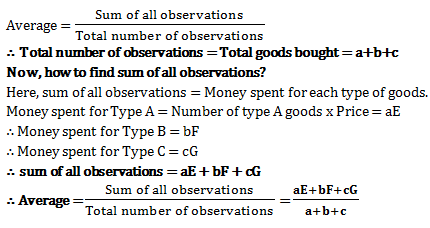# Average - Quantitative Aptitude (MCQ) questions for Q. 29282

Q.  Which of the following exactly denotes the average price of all the goods together if, Ramesh buys ‘a’ number of goods of type ‘A’ at price of Rs. ‘E’ each, ‘b’ number of goods of type ‘B’ at price of Rs. ‘F’ each and ‘c’ number of goods of type ‘C’ at price of Rs. ‘G’ each?
- Published on 11 Apr 17

a. (E+F+G) / (a+b+c)
b. (AE+BF+CG) / (a+b+c)
c. (aE+bF+cG) / (a+b+c)
d. (aA+bB+cC) / (a+b+c)